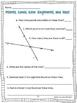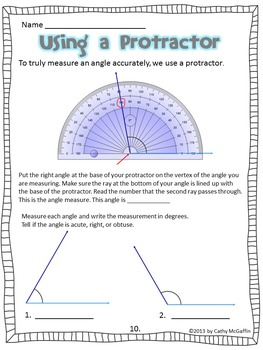Geometry Printables: Lines and AnglesSubject
Resource Type
Product Rating
File Type

PDF (Acrobat) Document File

Be sure that you have an application to open this file type before downloading and/or purchasing.

3 MB|29 plus answer key
Share
Also included in:
1. This file consists of my Smart Board Geometry Lines and Angles file and my Lines and Angles printables bundled together. When used together, they are a powerful way to help your students understand all the various lines and angles, and learn how to use a protractor. Topics covered in these interac
\$12.00
\$9.50
Save \$2.50
Product Description
These printables correlate perfectly with my Geometry SMARTBoard File: Lines and Angles , and if you have a SMARTBoard, you may want to consider Geometry SMARTBoard and Printables Bundle: Lines and Angles but even without a SMARTBoard, they are a great way to support your instruction of Common Core geometry and measurement standards. I created and used this file with my 4th grade students with great success, but you could also use it as review in 5th grade.

This 26 page file will provide an overview of the line and angle vocabulary expected at the 4th grade level. There is an assessment at the end. Answer keys are included for all pages.

Topics Covered:
• Points, lines, line segments, rays
• Types of Lines: parallel, intersecting, perpendicular
• Review of vertical, horizontal, and diagonal lines
• Types of Angles: acute, right, obtuse, straight
• How to use a protractor to measure angles
• How to use a protractor to sketch angles of a given measure
• How to use a landmark angle measure, such as 90° to estimate other angle measures
• Finding missing angle measures

Common Core Standards Addressed:
Geometry:
• 4.G.A.1 Draw points, lines, line segments, rays, angles (right, acute, obtuse), and perpendicular and parallel lines. Identify these in two-dimensional figures.
Geometric measurement: understand concepts of angle and measure angles.
• 4.MD.C.5 Recognize angles as geometric shapes that are formed wherever two rays share a common endpoint, and understand concepts of angle measurement:
• 4.MD.C.5a An angle is measured with reference to a circle with its center at the common endpoint of the rays, by considering the fraction of the circular arc between the points where the two rays intersect the circle. An angle that turns through 1/360 of a circle is called a “one-degree angle,” and can be used to measure angles.
• 4.MD.C.5b An angle that turns through n one-degree angles is said to have an angle measure of n degrees.
• 4.MD.C.6 Measure angles in whole-number degrees using a protractor. Sketch angles of specified measure.
• 4.MD.C.7 Recognize angle measure as additive. When an angle is decomposed into non-overlapping parts, the angle measure of the whole is the sum of the angle measures of the parts. Solve addition and subtraction problems to find unknown angles on a diagram in real world and mathematical problems, e.g., by using an equation with a symbol for the unknown angle measure.

I recommend beginning your study of geometry with Lines and Angles and then moving on to polygons. That way, when you teach polygons, your students will have the necessary vocabulary to classify them by attributes of lines and angles. Check out my Polygon Files.
Geometry SMARTBoard and Printables Bundle: Polygons

Geometry SMARTBoard File: Polygons

Geometry Printables: Polygons

I also have sets of Geometry Vocabulary Cards for both Lines & Angles and Polygons.
Geometry Vocabulary Cards for Lines and Angles
Geometry Vocabulary Cards for Polygons

Thanks for your interest in my product.
Please let me know if you have any
questions!
Cathy, aka Downeast Teach
Total Pages
29 plus answer key
Included
Teaching Duration
N/A
Report this Resource to TpT
Reported resources will be reviewed by our team. Report this resource to let us know if this resource violates TpT’s content guidelines.